《金哥百变Python零基础教程0》~变量与运算

# 本章知识点 :> `变量与运算`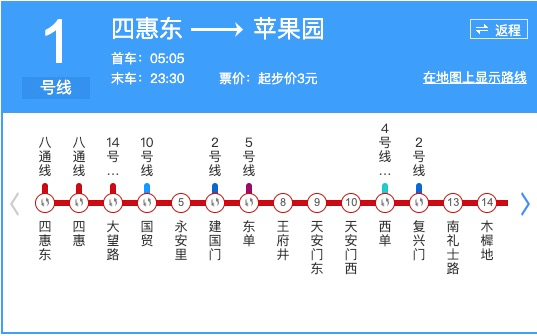# ：》那么学Python的秘诀是什么呢?

but仔细回想一下，这三个能力也不是天生的（反而还很难学哦~）。

`a-` -> `a^a^` -> `da` -> `da~da~` -> `j~j~` -> `ba~ba~` -> `ma~ma~` 我们发现一个baby学任何东西的秘诀：就是把一件`简单`的事`重复`的做（做几百上千次)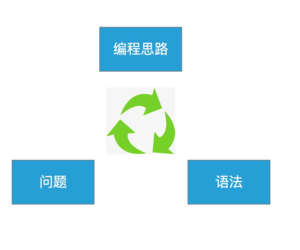# 有人会说了，“切~就这！还用你说"。# 变量与运算

• 变量和运算总要在一起才有意义

• 先看数字类的

• `a = 100 #定义一个数字a，值为100`
`b = a + a #定义一个数字b，值为a+a, 即200`
`pi = 3.14 #定义一个数字pi，值为3.14（浮点数)`
`c = 9 * 9 #定义一个数字c，值为9乘以9, 值为81`
`年龄 = 20 #定义一个数字 年龄，值为20`
`print(c) #打印数字c（屏幕打印)`
`print(1+2*3) #打印1+2*3的结果（将打印7)`
试一下 在线Python
• 再看文本类的（字符串）

• `s = 'hello world' #定义一个字符串s，值为 hello world`
`s1 = "hello world" #定义一个字符串s1（双引号)，值为 hello world`
`s2 = '中国人' #定义一个字符串s2，值为 中国人`
`print(s) #打印字符串s （屏幕打印)`
`l = len(s) #定义一个数字l，用len计算s的长度(有几个字符），l的值为11`
`名字 = "金哥" #定义一个字符串 名字，值为 金哥`
试一下 在线Python
• 信息量有点大，好在每一句单独看都不算难。

• 先解释一下 ·#· 这个符号，有没有发现在上面的代码里，每一个语句都有个 ·#·号
• ·#· 在Python里表示注释，就是用中文来解释一行（或一段）代码，所以
`a = 100 #定义一个数字a，值为100`
`a = 100 #定义一个数字a，让它等于100`
`a = 100`
• 以上3行代码的意义完全一样（#后面的中文怎么写，写不写都不影响代码本身的意义)

• 如果我们将#后的中文视为问题，左边的代码就是它的解
• 问题：定义一个数字a，值为100
• 解：`a = 100`
• 这就是一个最基础的 `简单重复` 动作
试一下 在线Python

# 具体有什么规律，规则呢？

• 我们先从 变量的角度说一下
• 所有包含 `定义xxx` 的代码，都需要取一个`变量`的名字，名字可以是字母 `a` `b` 字母加数字 `s1` `s2` 中文 `年龄` `名字`
• 每一个`变量`必须有一个`值`, 不写也有默认值
• `a = 100`
• 我们用 `=` 来进行变量的`赋值`,其实就是把右边的 `变量` `100` 赋值 给`变量` `a`
• 所以,下面的代码：
• `b = a`
• 此时又定义了一个`变量` `b`, 它的值是多少?(为a，也就是100）
试一下 在线Python

• 定义一个字符串` 'hello world' ` 和定义数字的方法是类似的
• `s = 'hello world'`
• 因为字符串（由字母组成）毕竟和数字不一样，所以把数字和字符串归为2个类型
• 数字 和 字符串，数字又可以分为 整型 `1` `2` 和 浮点型 `3.14`
• Python代码里出现的数字都是数字类型，出现的字符用引号的 `''` `""` 都是字符串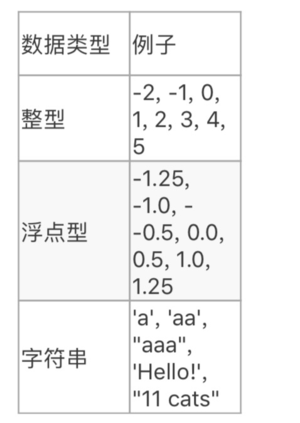试一下 在线Python

• 易错点：字符串的 `'``"` 要用英文，不能用中文引号
• 易错点：字符串必须带 引号，不带会被识别成变量名
• `名字 = '金哥'`
`print(’名字‘)`
`print(名字)`

试一下 在线Python
• 易错点：字符串`'123'` 和 数字 `123` 是两个类型的(特性不同，后面会细说)

• 我们再从运算的角度说一下
• 先说一个基本定律：`在Python代码里，出现的所有东西不是 变量 就是 运算符`
• 学习python首先就是要学会识别代码里的符号是 `变量` 还是 `运算`
`a = 100 `
`b = a + a `
`pi = 3.14`
`c = 9 * 9`
`年龄 = 20 `
`print(c)`
`print(1+2*3)`
`s = 'hello world'`
`s1 = "hello world"`
`s2 = '中国人'`
`print(s)`
`l = len(s)`
`名字 = "金哥"`
• 变量：`a` `100` `b` `pi` `3.14` `c` `9` `年龄` `20` `1` `2` `3` `s` `hello world` `s1` `s2` `中国人` `l` `名字` `金哥`
• 运算：`=` `+` `*` `print` `len`
• 每个运算有自己的含义，用于解特定问题，运算一定是计算`变量`
• `变量` 运算完的结果还是 `变量`
`a = 100 `
`b = a + a `
• 这里的 + 是数字相加，a + a 是数字a 加 数字 a得到的结果是一个变量，值是200
• 然后才是把这个200赋值给b
• 所以，多个运算可以不断连接在一起
`a = 100 `
`a + a`
• 这个例子里，没有变量b，但是a+a仍然会运算
试一下 在线Python

• `+ - * / (加 减 乘 除)` 可以做数字的运算
• 也可以连起来运算 `1+2*3`
• `+` 可以用于字符串相加
• `'abc‘ + ’def'` 结果是 `'abcdef'`
• `*` 可以用于字符串乘以数字
• `'abc' * 3` 结果是 `'abcabcabc'`
• 还有其他运算：

•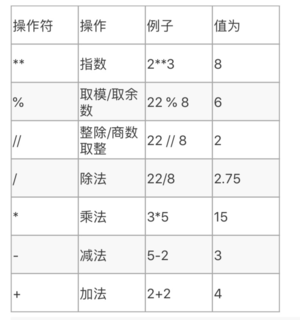试一下 在线Python

• 现在说一下 `print` 运算（当然，print这种学名是 函数，这个后面细讲)
• `print(变量)` 意思就是把`变量` 打印到 屏幕
• 没错，print可以打印任意变量
• `print(c)`
`print(1+2*3)`
`print(s)`
`print(print)`
• 你看的没错，甚至可以`print`打印`print`
试一下 在线Python

• 说一下`len` ; 作用：计算字符串长度（还能算其他变量的长度后面细讲)
• `print(len("abc"))`
• 你一定要逐渐习惯这样，把多个运算连起来的表达方式~
试一下 在线Python

# 综合运用• 问题：我们简化一下1号线地铁问题，假设只为 王府井（8号站）写一个Python程序，再假设问路人都是往 西单方向的（右边），思考下怎么写？（复杂一点的现有知识还做不了，后面会再细讲)

• 思路：

• 一切皆是 `变量` + `运算`, Python编程思路的第一步，把我们这个问题的解决方案看做一个新的运算
• 假设我们已经写出1段代码解这个问题，我们把这段代码合起来看做一个运算，暂时叫 `”王府井右行乘客问路回答算法“ `
• 所谓思路其实就是把 `”王府井右行乘客问路回答算法“ ` 进行分解，分解到 `数字` `字符串` `+ - * /` 基础运算
• `”王府井右行乘客问路回答算法“ ` 得输入变量是什么? 输出变量是什么？一般思路都是这么开始！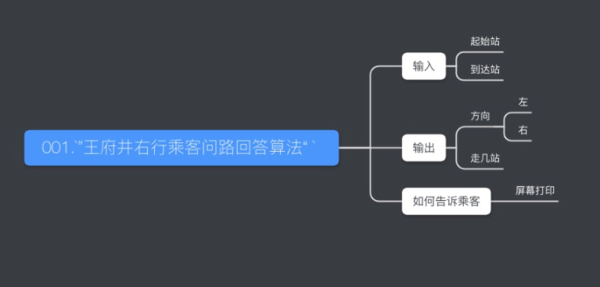• 现在我们把所有的变量，确定到类型，并尝试思考 输入如何计算成输出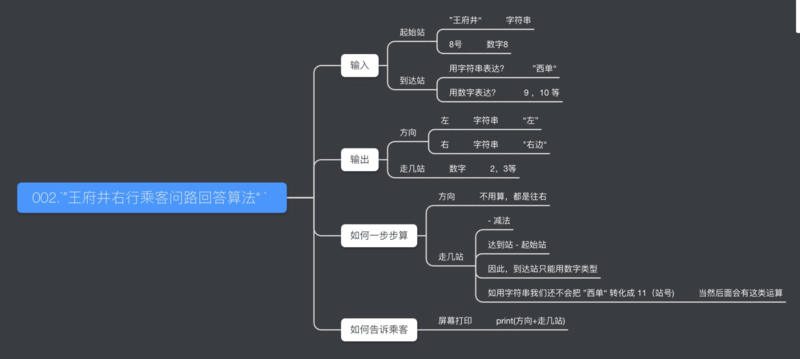• 现在可以把所有的变量和运算化成代码实现了

`起始站 = '王府井'`
`起始站号 = 8`
`到达站 = '西单'`
`达到站号 = 11`
`方向 = '右'`
`走几站 = 到达站号 - 起始站号`
`print('往' + 方向 + ‘走’)`
`print(走几站)`
`print('站‘)`
• 不习惯变量名用中文，也可以这么写

`start = '王府井'`
`start_num = 8`
`end = '西单'`
`end_num = 11`
`fangxiang = '右'`
`zou_num = end_num - start_num`
`print('往' + fangxiang + ‘走’)`
`print(zou_num)`
`print('站‘)`

试一下 在线Python

# 下一章会先复习 `变量` 与 `运算` 的知识，不用担心今天的新知识太多~

• p0总结. p0总结在线看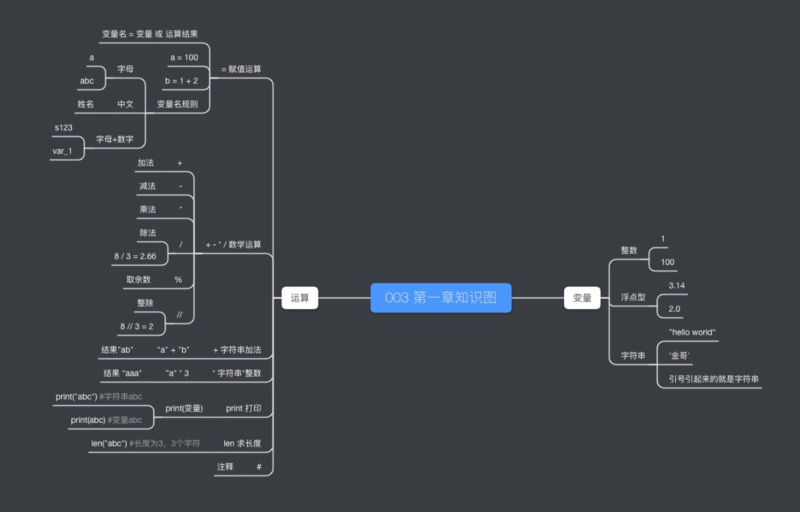Sorry but the SQLBolt exercises require a more recent browser to run.